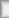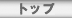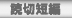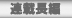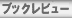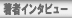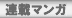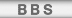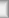# \$B\$\\$/\$O\$=\$&!"\$\\$/\$@\$C\$?!#\$"\$k\$\$\$O\$=\$&\$@\$C\$?\$b\$N!#(J

I was me. Or what it was.

## \$B>.NS\$R\$m\$-(J

\$B7G:\9f(J
\$B%?%\$%H%k(J
HTML
\$BBh#1#7#39f(J
\$B\$\\$/\$O\$=\$&!"\$\\$/\$@\$C\$?!#\$"\$k\$\$\$O\$=\$&\$@\$C\$?\$b\$N!#(J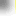\$B%H%C%W(J\$B!?(J\$BFI@ZC;JT(J\$B!?(J\$BO":\D9JT(J\$B!?(J\$B%3%i%`(J
\$B%V%C%/%l%S%e!<(J\$B!?(J\$BCx\$B!?(J\$BO":\%^%s%,(J\$B!?(JBBS\$B!?(J\$BJT=8ItF|5-(J
\$BCx:n8"(J\$B!?(J\$B%W%i%\$%P%7!<%]%j%7!<(J\$B!?(J\$B%5%\$%H%^%C%W(J